Courses

# Environmental Engineering 1

## 10 Questions MCQ Test RRB JE for Civil Engineering | Environmental Engineering 1

Description
This mock test of Environmental Engineering 1 for Civil Engineering (CE) helps you for every Civil Engineering (CE) entrance exam. This contains 10 Multiple Choice Questions for Civil Engineering (CE) Environmental Engineering 1 (mcq) to study with solutions a complete question bank. The solved questions answers in this Environmental Engineering 1 quiz give you a good mix of easy questions and tough questions. Civil Engineering (CE) students definitely take this Environmental Engineering 1 exercise for a better result in the exam. You can find other Environmental Engineering 1 extra questions, long questions & short questions for Civil Engineering (CE) on EduRev as well by searching above.
QUESTION: 1

### 1 TCU is equivalent to the color produced by

Solution:

TCU represent the true colour unit. It is a unit to represent colour impurities in water. 1 TCU is equivalent to the colour produced by 1 mg/L of platinum in form of chloroplatinate ion.

QUESTION: 2

### As per IS 10500:2012, the total concentration of manganese (as Mn) and iron (as Fe) for drinking water purpose shall not exceed

Solution:

As per IS10500:2012, the total concentration of manganese (as Mn) and iron (as Fe) for drinking water purpose shall not exceed 0.3 mg/L.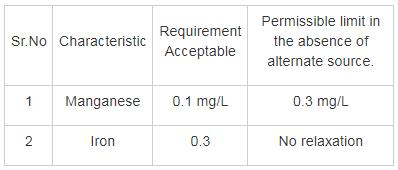QUESTION: 3

### Answer using the codes given below the list:-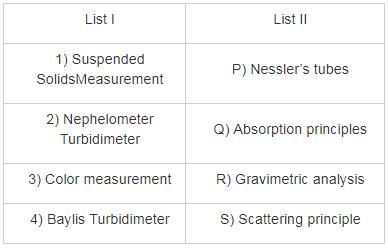Solution:

1) Measurement suspended solid is done by a gravimetric test involving the mass of residual measurement.

2) Measurement of Turbidity: -

A) Based on absorption principles

i) Turbidity Rod

ii) Jackson’s Turbidity meter

iii) Baylis Turbidimeter

B) Based on the scattering principle

i) Modern Nephelometer

3) Through Nessler’s tubes on Burgess scale, true colour is measured.

QUESTION: 4

The total domestic water demand for average Indian people is:

Solution: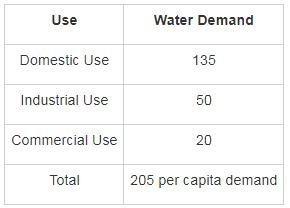QUESTION: 5

Two samples of water form two different sources are collected to measure pH value and it was found to be 6.2 and 6.8. How many times the first sample is more acidic than the second sample?

Solution:

Concept:-

pH = -log10 [H+]

-log10 [H+] = pH

[H+] = 10 -pH

Calculation

[H+]I = 10-6.2

[H+]II = 10-6.8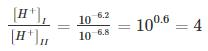Hence, first sample is 4 times acidic than second sample.

QUESTION: 6

Match the following:-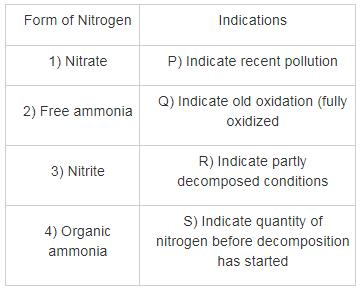Solution:

Free Ammonia – indicate recent pollution

Nitrate – indicate old pollution (fully oxidized)

Nitrite – indicate partly decomposed condition

Organic – indicate quantity of nitrogen before decomposition started.

QUESTION: 7

A water sample analysis produces alkalinity and total hardness of 200 mg/L and 250 mg/L as CaCO3 respectively. The carbonate and non-carbonate hardness (as CaCO3) will respectively be

Solution:

Concept:

Carbonate hardness = minimum of {Alkalinity, Total Hardness}

Total hardness = Carbonate Hardness + Non-carbonate Hardness.

Calculation:

Carbonate Hardness= minimum of {200 mg/L , 250 mg/L}

Carbonate Hardness = 200 mg/L as CaCO3

Non-Carbonate Hardness = Total Hardness – Carbonate Hardness

= 250 – 200

= 50 mg/L ​as CaCO3

QUESTION: 8

The fire demand for a city of 150000 population by using Kuichling’s formula is

Solution:

Concept:

Calculating fire Demand:

a) Kuchling’s formula

Q = 3182 √p

Where, Q = Amount of water required in liter/minute.

P = Population in Thousand

b) Freeman’s formula: -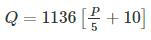Where, Q = Amount of water required in liter/minute.

P = Population in Thousand

c) National Bard of fire under writer formula:-

i. For central congested High valued city

→ when population ≤ 2 lakhs

Q = 4637 √ p [1 – 0.01 √p]

Where, Q = Amount of water required in liter/minute.

P = Population in Thousand

→ when population > 2 lakhs.

Provision of 54000 liters/minute may be made with an extra additional provision of 9100 to 36000 liter/minute for a second fire.

ii) for residential Buildings

→ Small or low building = 2200 l/min

→ Larger or higher building = 4500 l/min

→ High valued residency = (7650 – 13500) l/min

→ Three story building

In densely built up area = up to 27000 l/min

d) Buston’s Formula: -

Q = 5663√ p

Calculation: -

According to Kuchling’s formula the fire demand is

Q = 3182 √P

Q = 3182 √150 L/min

Q = 38971.38 L/min

Q = 56.12 MLD ~ 57 MLD

QUESTION: 9

Match List – I (Estimated method) with List – II (Corresponding indicator) and select the correct answer using the codes given below the lists: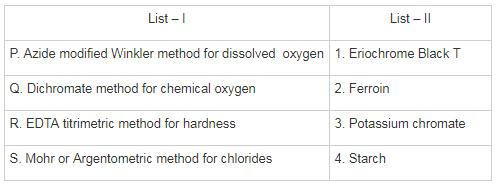Solution: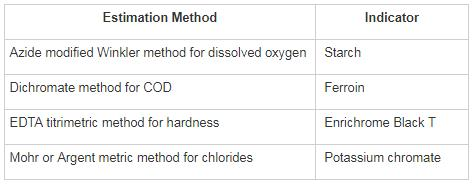QUESTION: 10

Which one of the following related to domestic potable water quality is CORRECT?

Solution:

Portable water quality parameter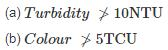(c) Hardness ϵ (75 to 115) ppm
(d) BOD = 0 ppm